factor command in Linux is used to print the prime factor of any given number. In this tutorial, you will learn factor command in Linux/Unix and uses of factor command with example.

Prime factor: A factor which is a prime number and not a composite number is a prime factor.

Prime Number: A number that can be divided exactly only by itself and 1.

Example: 3, 5, 7, 13

Factor: A factor is a number that divides another number completely with no remainder.

Prime Factorization: Prime factorization is a way of expressing a number as a product of its prime factors.

## Syntax for factor command

`factor number`
`factor option`

Use the command factor –help to get help for the factor command and know the option available for factor command.

`factor --help`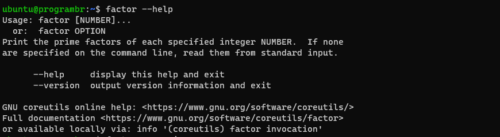Use factor –version command to know the current version of factor command line utility.

`factor --version`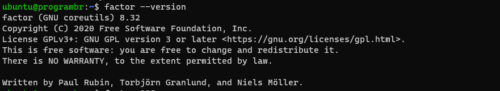## Finding the factor of a single number

Using the factor command you can display the prime factor of any number. Follow the below syntax for finding the factor of any number.

`factor Number`

Do not forget to replace the Number with your desired number for finding factor.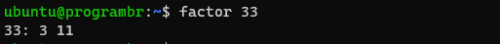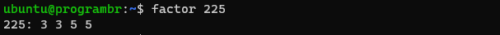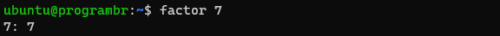## Finding Factor of multiple numbers

Using the factor command you can display the prime factor of more than one number at once. Follow the below syntax for finding the factor of multiple numbers at once.

`factor numb1 numb2 numb3 numb4 .....`

Do not forget to replace numb1 numb2 numb3 numb4 with your desired numbers for finding factor.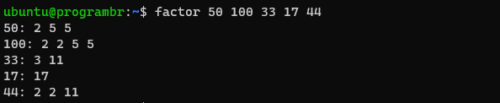Sharing is Caring
Scroll to Top